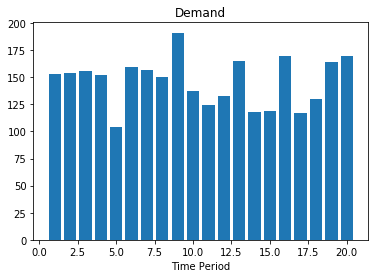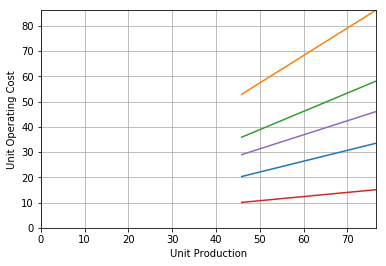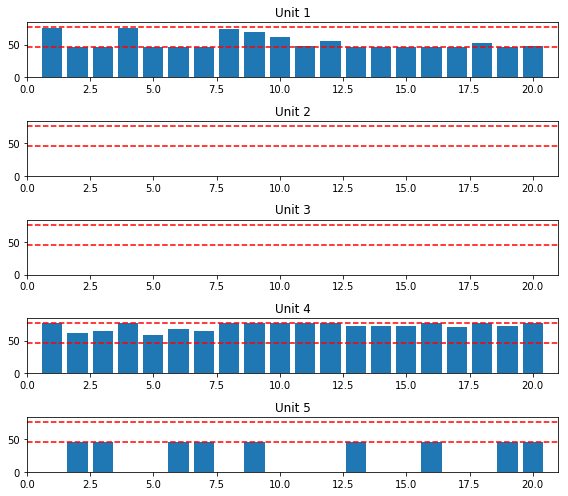This notebook contains material from ND-Pyomo-Cookbook; content is available on Github. The text is released under the CC-BY-NC-ND-4.0 license, and code is released under the MIT license.

# 4.6 Unit Commitment¶

Keywords: semi-continuous variables, cbc usage, gdp, disjunctive programming

## 4.6.1 Imports¶

In :
%matplotlib inline
import matplotlib.pyplot as plt
import numpy as np
import pandas as pd
from IPython.display import display, HTML

import shutil
import sys
import os.path

if not shutil.which("pyomo"):
!pip install -q pyomo
assert(shutil.which("pyomo"))

if not (shutil.which("cbc") or os.path.isfile("cbc")):
!apt-get install -y -qq coinor-cbc
else:
try:
!conda install -c conda-forge coincbc
except:
pass

assert(shutil.which("cbc") or os.path.isfile("cbc"))
import pyomo.environ as pyo
import pyomo.gdp as gdp


## 4.6.2 Problem statement¶

A set of $N$ electrical generating units are available to meet a required demand $d_t$ for time period $t \in 1, 2, \ldots, T$. The power generated by unit $n$ for time period $t$ is denoted $x_{n,t}$. Each generating unit is either off, $x_{n,t} = 0$ or else operating in a range $[p_n^{min}, p_n^{max}]$. The incremental cost of operating the generator during period $t$ is $a_n x_{n,t} + b_n$. A binary variable variable $u_{n,t}$ indicates the operational state of a generating unit.

The unit commmitment problem is then

\begin{align*} \min \sum_{n\in N} \sum_{t\in T} a_n x_{n,t} + b_n u_{n,t} \end{align*}

subject to

\begin{align*} \sum_{n\in N} x_{n,t} & = d_t \qquad \forall t \in T \\ p_{n}^{min}u_{n,t} & \leq x_{n,t} \qquad \forall n \in N, \ \forall t \in T \\ p_{n}^{max}u_{n,t} & \geq x_{n,t} \qquad \forall n \in N, \ \forall t \in T \\ \end{align*}

where we use the short-cut notation $T = [1, 2, \ldots T]$ and $N = [1, 2, \ldots, N]$.

This is a minimal model. A realistic model would include additional constraints corresponding to minimum up and down times for generating units, limits on the rate at which power levels can change, maintenance periods, and so forth.

## 4.6.3 Model¶

### 4.6.3.1 Demand¶

In :
# demand
T = 20
T = np.array([t for t in range(0, T)])
d = np.array([100 + 100*np.random.uniform() for t in T])

fig, ax = plt.subplots(1,1)
ax.bar(T+1, d)
ax.set_xlabel('Time Period')
ax.set_title('Demand')

Out:
Text(0.5, 1.0, 'Demand')### 4.6.3.2 Generating Units¶

In :
# generating units
N = 5
pmax = 2*max(d)/N
pmin = 0.6*pmax

N = np.array([n for n in range(0, N)])
a = np.array([0.5 + 0.2*np.random.randn() for n in N])
b = np.array([10*np.random.uniform() for n in N])

p = np.linspace(pmin, pmax)

fig, ax = plt.subplots(1,1)
for n in N:
ax.plot(p, a[n]*p + b[n])
ax.set_xlim(0, pmax)
ax.set_ylim(0, max(a*pmax + b))
ax.set_xlabel('Unit Production')
ax.set_ylabel('Unit Operating Cost')
ax.grid()### 4.6.3.3 Pyomo model 1: Conventional implementation for emi-continuous variables¶

In :
def unit_commitment():
m = pyo.ConcreteModel()

m.N = pyo.Set(initialize=N)
m.T = pyo.Set(initialize=T)

m.x = pyo.Var(m.N, m.T, bounds = (0, pmax))
m.u = pyo.Var(m.N, m.T, domain=pyo.Binary)

# objective
m.cost = pyo.Objective(expr = sum(m.x[n,t]*a[n] + m.u[n,t]*b[n] for t in m.T for n in m.N), sense=pyo.minimize)

# demand
m.demand = pyo.Constraint(m.T, rule=lambda m, t: sum(m.x[n,t] for n in N) == d[t])

# semi-continuous
m.lb = pyo.Constraint(m.N, m.T, rule=lambda m, n, t: pmin*m.u[n,t] <= m.x[n,t])
m.ub = pyo.Constraint(m.N, m.T, rule=lambda m, n, t: pmax*m.u[n,t] >= m.x[n,t])
return m

m = unit_commitment()
pyo.SolverFactory('cbc').solve(m).write()

fig, ax = plt.subplots(max(N)+1, 1, figsize=(8, 1.5*max(N)+1))
for n in N:
ax[n].bar(T+1, [m.x[n,t]() for t in T])
ax[n].set_xlim(0, max(T)+2)
ax[n].set_ylim(0, 1.1*pmax)
ax[n].plot(ax[n].get_xlim(), np.array([pmax, pmax]), 'r--')
ax[n].plot(ax[n].get_xlim(), np.array([pmin, pmin]), 'r--')
ax[n].set_title('Unit ' + str(n+1))
fig.tight_layout()

# ==========================================================
# = Solver Results                                         =
# ==========================================================
# ----------------------------------------------------------
#   Problem Information
# ----------------------------------------------------------
Problem:
- Name: unknown
Lower bound: 1018.71533244
Upper bound: 1018.71533244
Number of objectives: 1
Number of constraints: 200
Number of variables: 180
Number of binary variables: 100
Number of integer variables: 100
Number of nonzeros: 180
Sense: minimize
# ----------------------------------------------------------
#   Solver Information
# ----------------------------------------------------------
Solver:
- Status: ok
User time: -1.0
System time: 0.06
Wallclock time: 0.06
Termination condition: optimal
Termination message: Model was solved to optimality (subject to tolerances), and an optimal solution is available.
Statistics:
Branch and bound:
Number of bounded subproblems: 0
Number of created subproblems: 0
Black box:
Number of iterations: 0
Error rc: 0
Time: 0.07869720458984375
# ----------------------------------------------------------
#   Solution Information
# ----------------------------------------------------------
Solution:
- number of solutions: 0
number of solutions displayed: 0### 4.6.3.4 Pyomo model 2: GDP implementation¶

In :
def unit_commitment_gdp():
m = pyo.ConcreteModel()

m.N = pyo.Set(initialize=N)
m.T = pyo.Set(initialize=T)

m.x = pyo.Var(m.N, m.T, bounds = (0, pmax))

# demand
m.demand = pyo.Constraint(m.T, rule=lambda m, t: sum(m.x[n,t] for n in N) == d[t])

# representing the semicontinous variables as disjuctions
m.sc1 = gdp.Disjunct(m.N, m.T, rule=lambda d, n, t: d.model().x[n,t] == 0)
m.sc2 = gdp.Disjunct(m.N, m.T, rule=lambda d, n, t: d.model().x[n,t] >= pmin)
m.sc = gdp.Disjunction(m.N, m.T, rule=lambda m, n, t: [m.sc1[n,t], m.sc2[n,t]])

# objective. Note use of the disjunct indicator variable
m.cost = pyo.Objective(expr = sum(m.x[n,t]*a[n] + m.sc2[n,t].indicator_var*b[n] for t in m.T for n in m.N), sense=pyo.minimize)

# alternative formulation. But how to access the indicator variable?
#m.semicontinuous = gdp.Disjunction(m.N, m.T, rule=lambda m, n, t: [m.x[n,t]==0, m.x[n,t] >= pmin])
pyo.TransformationFactory('gdp.chull').apply_to(m)
return m

m_gdp = unit_commitment_gdp()
pyo.SolverFactory('cbc').solve(m_gdp).write()

# ==========================================================
# = Solver Results                                         =
# ==========================================================
# ----------------------------------------------------------
#   Problem Information
# ----------------------------------------------------------
Problem:
- Name: unknown
Lower bound: 863.60019688
Upper bound: 863.60019688
Number of objectives: 1
Number of constraints: 20
Number of variables: 100
Number of binary variables: 200
Number of integer variables: 200
Number of nonzeros: 100
Sense: minimize
# ----------------------------------------------------------
#   Solver Information
# ----------------------------------------------------------
Solver:
- Status: ok
User time: -1.0
System time: 0.02
Wallclock time: 0.03
Termination condition: optimal
Termination message: Model was solved to optimality (subject to tolerances), and an optimal solution is available.
Statistics:
Branch and bound:
Number of bounded subproblems: 0
Number of created subproblems: 0
Black box:
Number of iterations: 0
Error rc: 0
Time: 0.04323315620422363
# ----------------------------------------------------------
#   Solution Information
# ----------------------------------------------------------
Solution:
- number of solutions: 0
number of solutions displayed: 0


### 4.6.3.5 There is a problem here!¶

Why are the results different? Somehow it appears values of the indicator variables are being ignored.

In :
for n in N:
for t in T:
print("n = {0:2d}  t = {1:2d}   {2} {3}  {4:5.2f}".format(n, t, m_gdp.sc1[n,t].indicator_var(), m_gdp.sc2[n,t].indicator_var(), m.x[n,t]()))

n =  0  t =  0   1.0 0.0  76.13
n =  0  t =  1   1.0 0.0  45.86
n =  0  t =  2   1.0 0.0  45.86
n =  0  t =  3   1.0 0.0  75.96
n =  0  t =  4   1.0 0.0  45.86
n =  0  t =  5   1.0 0.0  45.86
n =  0  t =  6   1.0 0.0  45.86
n =  0  t =  7   1.0 0.0  73.80
n =  0  t =  8   1.0 0.0  68.79
n =  0  t =  9   1.0 0.0  61.04
n =  0  t = 10   1.0 0.0  47.89
n =  0  t = 11   1.0 0.0  56.14
n =  0  t = 12   1.0 0.0  45.86
n =  0  t = 13   1.0 0.0  45.86
n =  0  t = 14   1.0 0.0  45.86
n =  0  t = 15   1.0 0.0  47.08
n =  0  t = 16   1.0 0.0  45.86
n =  0  t = 17   1.0 0.0  53.14
n =  0  t = 18   1.0 0.0  45.86
n =  0  t = 19   1.0 0.0  47.56
n =  1  t =  0   1.0 0.0   0.00
n =  1  t =  1   1.0 0.0   0.00
n =  1  t =  2   1.0 0.0   0.00
n =  1  t =  3   1.0 0.0   0.00
n =  1  t =  4   1.0 0.0   0.00
n =  1  t =  5   1.0 0.0   0.00
n =  1  t =  6   1.0 0.0   0.00
n =  1  t =  7   1.0 0.0   0.00
n =  1  t =  8   1.0 0.0   0.00
n =  1  t =  9   1.0 0.0   0.00
n =  1  t = 10   1.0 0.0   0.00
n =  1  t = 11   1.0 0.0   0.00
n =  1  t = 12   1.0 0.0   0.00
n =  1  t = 13   1.0 0.0   0.00
n =  1  t = 14   1.0 0.0   0.00
n =  1  t = 15   1.0 0.0   0.00
n =  1  t = 16   1.0 0.0   0.00
n =  1  t = 17   1.0 0.0   0.00
n =  1  t = 18   1.0 0.0   0.00
n =  1  t = 19   1.0 0.0   0.00
n =  2  t =  0   1.0 0.0   0.00
n =  2  t =  1   1.0 0.0   0.00
n =  2  t =  2   1.0 0.0   0.00
n =  2  t =  3   1.0 0.0   0.00
n =  2  t =  4   1.0 0.0   0.00
n =  2  t =  5   1.0 0.0   0.00
n =  2  t =  6   1.0 0.0   0.00
n =  2  t =  7   1.0 0.0   0.00
n =  2  t =  8   1.0 0.0   0.00
n =  2  t =  9   1.0 0.0   0.00
n =  2  t = 10   1.0 0.0   0.00
n =  2  t = 11   1.0 0.0   0.00
n =  2  t = 12   1.0 0.0   0.00
n =  2  t = 13   1.0 0.0   0.00
n =  2  t = 14   1.0 0.0   0.00
n =  2  t = 15   1.0 0.0   0.00
n =  2  t = 16   1.0 0.0   0.00
n =  2  t = 17   1.0 0.0   0.00
n =  2  t = 18   1.0 0.0   0.00
n =  2  t = 19   1.0 0.0   0.00
n =  3  t =  0   1.0 0.0  76.43
n =  3  t =  1   1.0 0.0  61.93
n =  3  t =  2   1.0 0.0  64.26
n =  3  t =  3   1.0 0.0  76.43
n =  3  t =  4   1.0 0.0  57.86
n =  3  t =  5   1.0 0.0  67.42
n =  3  t =  6   1.0 0.0  65.12
n =  3  t =  7   1.0 0.0  76.43
n =  3  t =  8   1.0 0.0  76.43
n =  3  t =  9   1.0 0.0  76.43
n =  3  t = 10   1.0 0.0  76.43
n =  3  t = 11   1.0 0.0  76.43
n =  3  t = 12   1.0 0.0  72.97
n =  3  t = 13   1.0 0.0  72.25
n =  3  t = 14   1.0 0.0  72.77
n =  3  t = 15   1.0 0.0  76.43
n =  3  t = 16   1.0 0.0  70.91
n =  3  t = 17   1.0 0.0  76.43
n =  3  t = 18   1.0 0.0  72.74
n =  3  t = 19   1.0 0.0  76.43
n =  4  t =  0   1.0 0.0   0.00
n =  4  t =  1   1.0 0.0  45.86
n =  4  t =  2   1.0 0.0  45.86
n =  4  t =  3   1.0 0.0   0.00
n =  4  t =  4   1.0 0.0   0.00
n =  4  t =  5   1.0 0.0  45.86
n =  4  t =  6   1.0 0.0  45.86
n =  4  t =  7   1.0 0.0   0.00
n =  4  t =  8   1.0 0.0  45.86
n =  4  t =  9   1.0 0.0   0.00
n =  4  t = 10   1.0 0.0   0.00
n =  4  t = 11   1.0 0.0   0.00
n =  4  t = 12   1.0 0.0  45.86
n =  4  t = 13   1.0 0.0   0.00
n =  4  t = 14   1.0 0.0   0.00
n =  4  t = 15   1.0 0.0  45.86
n =  4  t = 16   1.0 0.0   0.00
n =  4  t = 17   1.0 0.0   0.00
n =  4  t = 18   1.0 0.0  45.86
n =  4  t = 19   1.0 0.0  45.86